ML Aggarwal Class 6 Solutions for ICSE Maths Model Question Paper 1 acts as the best resource during your learning and helps you score well in your exams.

## ML Aggarwal Class 6 Solutions for ICSE Maths Model Question Paper 1

Choose the correct answer from the given four options (1-2):
Question 1.
If the sum of two integers is -21 and one of them is -10 then the other is
(a) -32
(b) 32
(c) -11
(d) 11
Solution: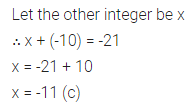Question 2.
The number of natural numbers between the smallest natural number and the greatest 2-digit number is
(a) 90
(b) 97
(c) 98
(d) 99
Solution: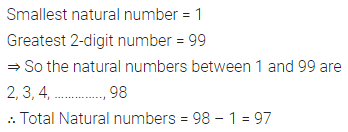Question 3.
Find the value of 25 × 37 × 8 × 6 by suitable arrangement.
Solution: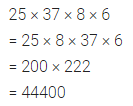Question 4.
Write four consecutive integers preceding -97.
Solution: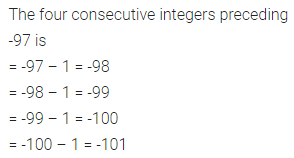Question 5.
Write the greatest and the smallest 4-digit numbers using four different digits with the condition that 5 occurs at ten’s place.
Solution:Question 6.
Write all possible natural numbers formed by the digits 7, 0 and 3. Repetition of digits is not allowed.
Solution:Question 7.
Find the value of: -237 – (-328) + (-205) – 76 + 89.
Solution: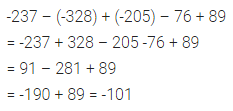Question 8.
Abhijeet’s school is 3 km 520 m away from his home. One day while returning from his school, just after covering 1 km 370 m distance, he saw a woman who was bleeding, he took her to the nearest hospital which was 2 km 775 m away from that place and got her admitted. He came back to his home which was 4 km 565 m from the hospital.
(i) Find the distance covered by Abhijeet on that day.
(ii) What value of life is depicted by Abhijeet?
Solution: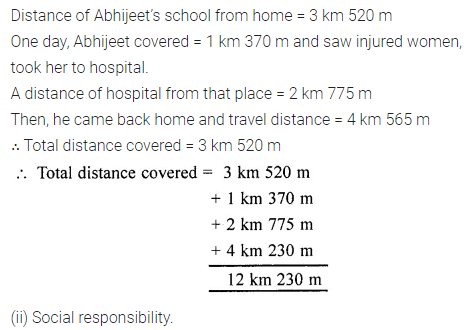Question 9.
Arrange the following integers in descending order:
-353, 207, -289, 702, -335, 0, -77.
Solution: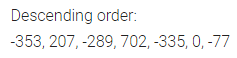Question 10.
Find the smallest five-digit number which is exactly divisible by 254.
Solution: